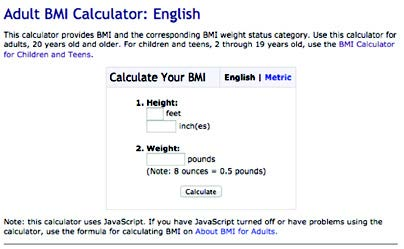# Does the audience have to conduct mathematical calculations?

Be sure to do the math rather than expect the audience to calculate. Provide calculations and conversions so that the audience isn’t distracted, confused or intimidated by the numbers and formulas or misinformed by errors in their calculations. Few people are likely to take the time or able to conduct even basic mathematical calculations such as addition and subtraction. Research shows health professionals and trained statisticians can make wrong assumptions and calculation errors, depending on the context and type of calculations involved.

Keep the denominators constant. When you use different denominators, people can’t easily compare numbers. Use the same denominator, even for absolute risk (example: 1 out of 3), throughout the material so that audiences don’t have to calculate.

### Example 1:This is an example of a tool that does the calculations rather than expect the audience to calculate the BMI themselves.

### Example 2:

How much money did you spend on alcohol last year?

If you had 3 alcoholic drinks a day and each drink cost \$4, then you spent \$4,380 last year on alcoholic drinks. What else could you do with \$4,380 this year?

Page last reviewed: August 7, 2019